# Using a net to find the surface area of a triangular prism Online Quiz

Following quiz provides Multiple Choice Questions (MCQs) related to Using a net to find the surface area of a triangular prism. You will have to read all the given answers and click over the correct answer. If you are not sure about the answer then you can check the answer using Show Answer button. You can use Next Quiz button to check new set of questions in the quiz.Q 1 - Find the surface area of the following triangular prism using net.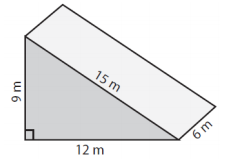### Explanation

Step 1:

Using net the surface area of triangular prism is

Sides of triangle base a = 9m; b = 12m; c = 15m; height h = 6m

Step 2:

Surface area of prism = 2 triangle area + h (a+b+c)

= 324 square m

Q 2 - Find the surface area of the following triangular prism using net.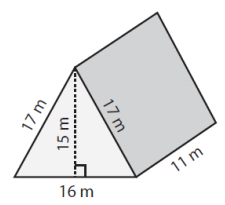### Explanation

Step 1:

Using net the surface area of triangular prism

Sides of triangle base a = 17m; b = 17m; c = 16m; height h = 11m

Step 2:

Surface area of prism = 2 triangle area + h (a+b+c)

= 790 square m

Q 3 - Find the surface area of the following triangular prism using net.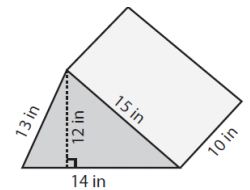### Explanation

Step 1:

Using net the surface area of triangular prism

Sides of triangle base a = 13in; b = 14in; c = 15in; height h = 10in

Step 2:

Surface area of prism = 2 triangle area + h (a+b+c)

= 588 square in

Q 4 - Find the surface area of the following triangular prism using net.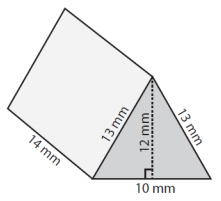### Explanation

Step 1:

Using net the surface area of triangular prism

Sides of triangle base a = 13mm; b = 13mm; c = 10mm; height h = 14mm

Step 2:

Surface area of prism = 2 triangle area + h (a+b+c)

= 624 square mm

Q 5 - Find the surface area of the following triangular prism using net.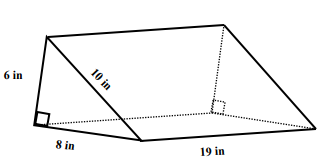### Explanation

Step 1:

Using net the surface area of triangular prism

Sides of triangle base a = 6in; b = 8in; c = 10in; height h = 19in

Step 2:

Surface area of prism = 2 triangle area + h (a+b+c)

= 504 square in

Q 6 - Find the surface area of the following triangular prism using net.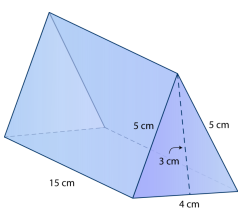### Explanation

Step 1:

Using net the surface area of triangular prism

Sides of triangle base a = 4cm; b = 5cm; c = 5cm; height h = 15cm

Step 2:

Surface area of prism = 2 triangle area + h (a+b+c)

= 228.33 square cm

Q 7 - Find the surface area of the following triangular prism using net.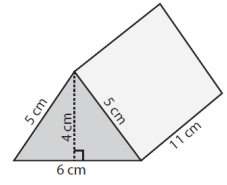### Explanation

Step 1:

Using net the surface area of triangular prism

Sides of triangle base a = 5cm; b = 5cm; c = 6; height h = 11cm

Step 2:

Surface area of prism = 2 triangle area + h (a+b+c)

= 200 square cm

Q 8 - Find the surface area of the following triangular prism using net.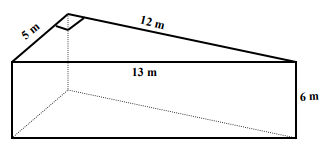### Explanation

Step 1:

Using net the surface area of triangular prism

Sides of triangle base a = 5m; b = 12m; c = 13m; height h = 6m

Step 2:

Surface area of prism = 2 triangle area + h (a+b+c)

= 240 square m

Q 9 - Find the surface area of the following triangular prism using net.### Explanation

Step 1:

Using net the surface area of triangular prism

Sides of triangle base a = 21cm; b = 28cm; c = 35cm; height h = 14cm

Step 2:

Surface area of prism = 2 triangle area + h (a+b+c)

= 1764 square cm

Q 10 - Find the surface area of the following triangular prism using net.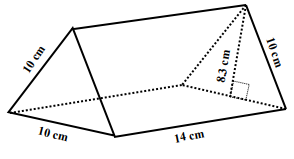### Explanation

Step 1:

Using net the surface area of triangular prism

Sides of triangle base a = 10cm; b = 10cm; c = 10cm; height h = 14cm

Step 2:

Surface area of prism = 2 triangle area + h (a+b+c)

= 506.6 square cm

using_net_to_find_surface_area_of_triangular_prism.htm